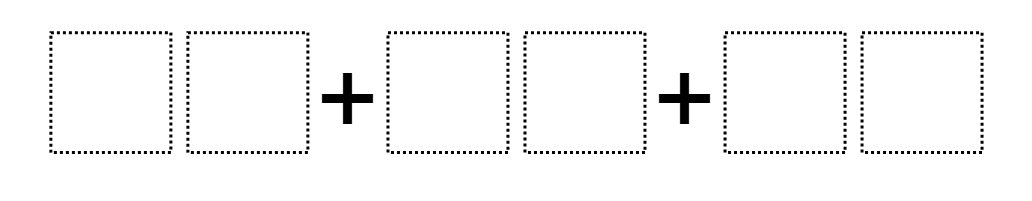Home > Grade 2 > Sums to 100

# Sums to 100

Directions: Using the digits 1 to 9 at most one time each, fill in the boxes to create the closest possible sum to 100.### Hint

Can you give me 3 numbers that add up to 100 (any number could be repeated)? How can we use this to fill in the boxes using each digit only once?

### Answer

Multiple solutions

59+24+17

Source: Graham Fletcher

## Close to 1000

Directions: Using the digits 1 to 9 at exactly one time each, fill in the …

### 7 comments

1.49 + 32 + 18 = 99

2.Our answer was 29+18+53=100

3.I had a lot of fun with this challenge and passed it along to my family when I couldn’t figure it out! Looking for more of these challenges in the future!

4.23+56+18=100

If I can get my students with behaviors to do this at 3 or upper I will challenge them to do this during morning warmup.

•23+56+18=97 not 100. It’s close though You might has meant 24+57+19=100

5.One of my classes came up with 42+38+19. It was great to see them put together the tens first, then they added all of the ones, and finally added the ones and tens for the final answer.